﻿ Development of a Computational Interface for Hydropower Plants

### Development of a Computational Interface for Hydropower Plants

Ajao K.R, Olabode O.F., Sule O.OPEN ACCESSPEER-REVIEWED

## Development of a Computational Interface for Hydropower Plants

Ajao K.R1,, Olabode O.F.1, Sule O.1

1Department of Mechanical Engineering, University of Ilorin, Ilorin, Nigeria

### Abstract

The computational interface for hydropower plants was developed with Microsoft Visual Studio 2010 and tested using some available data from Jebba hydropower station to validate its functionality due to non availability of data for small hydropower plants which the interface is designed for. Hitherto several other methods have been used in hydropower computation, these methods range from manual computation with the use of pen and paper to the use of Microsoft Excel spreadsheet, some others utilize Visual Basic to commercial software. This interface was designed to compute flow duration values, plant capacity, power duration and the available energy. The program also have the project report feature, where user can view a concise report of the computation, save and print report sheets.

### At a glance: Figures

12
Prev Next

• K.R, Ajao, Olabode O.F., and Sule O.. "Development of a Computational Interface for Hydropower Plants." Sustainable Energy 2.2 (2014): 63-80.
• K.R, A. , O.F., O. , & O., S. (2014). Development of a Computational Interface for Hydropower Plants. Sustainable Energy, 2(2), 63-80.
• K.R, Ajao, Olabode O.F., and Sule O.. "Development of a Computational Interface for Hydropower Plants." Sustainable Energy 2, no. 2 (2014): 63-80.

 Import into BibTeX Import into EndNote Import into RefMan Import into RefWorks

### 1. Introduction

Hydroelectric power is the power generated from the energy of falling water. When water fall due to gravity, the kinetic energy thereof can be converted to mechanical energy and then to electrical energy which is the most usable form of energy .

Small hydropower plant does not have a universal definition due to differences in what is accepted to be ‘small’ in different countries, but generally a small hydropower plant can be described to be a plant that can generate between 1MW to 50MW . Thus, any plant capacity above the stated value may be considered as large hydropower plant.

It is essential to make necessary computations to determine the operating conditions of any hydroelectric station. It is from such computation that some details of the plant such as plant capacity, flow duration values and curves, renewable energy available can be obtained .

Before a hydropower plant design and construction is fully started, there is a great need to obtain the flow duration data which is used to generate the flow duration curve, this is referred to as hydrological modeling , and the power duration curve is also of equal importance. At this stage the outcome of the calculation helps to determine whether the whole project is worthwhile or not, especially when it is compared with cost and power demand.

It is necessary to make hydropower calculations because:

i. It can be used to predict the expected output of a hydropower plant

ii. It is efficient in determining the future flow characteristics of the site

iii. For existing sites that are not performing optimally, the parameters can be checked to study why there is drop in output and how best to boost the output.

Furthermore the computational interface is even more important because:

i. It presents a fast and more accurate means of design calculation when compared with manual estimation which on the other hand involves dealing with large figures that can easily induce human error.

ii. It is possible to easily compare values to study which one gives a closer output to the desired output without having to go through the rigorous activity of manual calculation.

iii. It presents an organized printable report which will help in construction and is also useful for future reference.

### 2.Materials and Methods

The Hydropower computation modules development process follows the general way of developing applications in Visual Basic express edition . The process is as follows:

i. Planning the project: It is at this stage that the conceptual view of the program is gotten, its structure, target users and ultimately the purpose for which the program will be written.

ii. Creating the project: this is the creation of all files necessary for the application which is known as the project and when more than one is referred to, it is known as solution.

iii. Designing the User interface: it involves dragging various controls onto the design surface or form. Then the properties that define the appearance and behaviour of the form and the contents are set.

iv. Writing the code: this involves writing of the visual basic code that defines how the application behaves and interacts with the user.

v. Testing the code: it is possible to have bugs in the application, bugs are errors that disallow the application from producing desired results, it is from this stage that the application is tested and errors are removed or debugging.

vi. The program is then compiled in an executable format (.exe) extension which runs on windows operating system. The application is then ready for distribution.

Each interface has its own particular use, some of the interfaces are passive, and they neither accept data nor contribute to the actual computation, they are only necessary to aid the use of the application or give useful information about the application, while the other set of interfaces are active, they are indispensable during computation, without some of them, computations cannot be made while others aid the accuracy of the computations.

2.1. Description of Program Interface

There is a need for a deeper design understanding than the architectural design and configuration specification as it contains the procedural methods with which the inputs are transformed to output. The algorithm for the program is also developed, which contains step by step solution to the problem.

2.1.1. The Flow Interface

The flow form was designed to have a data grid view which displays data loaded into the application, it has two buttons, one for opening the open file dialog which helps in selecting the file to load while the other is the OK button for accepting the loaded data. The strength of this interface lies in its codes and after data has been loaded to the memory, they are sorted in descending order using the Bubble Sort Method , and a rank is given to each value, the ranking was programmed to give the same rank to equal values and skip the next rank depending on how many values are equal. This and the equation for calculating the percentage equaled or exceeded are available on the web . The equation for calculating percentage equaled or exceeded is given as:(1)

where,

P = the probability that a given flow will be equalled or exceeded (% of time)

M = the ranked position on the list

n = the number of events.

3.1.2. The Design Flow/Gross Head, Percentage Specified, Residual Flow And Losses Interface

All these forms are designed and function in the same way, they have textboxes into which the user enters the needed data, and a button which when clicked accepts the input data and stores at for use on other forms. Design flow is the maximum flow the turbine can use, the Gross head is the height of the falling water from the turbine, the percentage specified is a percentage value whose corresponding available flow value is the firm flow, and the residual flow is the flow left in the river throughout the year for environmental reasons. The losses include : transformer losses which is the loss that occur due to the matching of the generator voltage to that of the transmission line, this value varies from 1-2%, parasitic electricity losses which is the loss due to the use of some of the energy generated to power auxiliary equipments it varies from 1-3%, maximum hydraulic losses which is the energy that is lost as water flows through the water passages and varies from 2-7% for most small hydropower plants. The maximum tail water which is the maximum reduction in available gross head that will occur during time of high flows in the river and annual downtime losses which is the loss due to the plant downtime and it is used in the computation of annual renewable energy available, it varies from 4-6%.

2.1.3. The Turbine Types Interface

This interface is responsible for accepting the user input of the type of turbine used, it has six radio buttons for the following types of turbine: Francis, Kaplan, Propeller, Pelton, Turgo and Cross flow Turbines. It also has a textbox for accepting manual efficiency which is enabled if the user decides not to use the model calculated efficiency and checks the enter efficiency manually radio button, the form also has a textbox that accepts the generator efficiency and a combo box for number of Jets which is used in the efficiency computation of Pelton and Turgo turbines.

2.2. Design Calculations
2.2.1. Turbine Efficiency

The Formulae for calculating the efficiency of the turbines are as given below :

Reaction turbine runner size(2)

where: d = runner throat diameter in mQd = Design flow (flow at rated head and full gate opening in m3/s)

Specific speed(3)

where:

nq = specific speed on flow

k = 800 for Kaplan and propeller turbines

k = 600 for Francis turbine

H = rated head on turbine in m

for Francis turbine:

Specific speed adjustment to peak efficiency:(4)

Runner size adjustment to peak efficiency: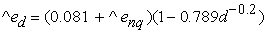(5)

Turbine peak efficiency:(6)

where:

Rm = turbine manufacture/design coefficient

Peak efficiency flow:(7)

Efficiencies at flows below peak efficiency flow: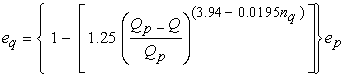(8)

Drop in efficiency at full load: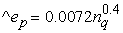(9)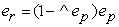(10)

Efficiencies at flows above peak efficiency flow: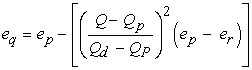(11)

for Kaplan and Propeller turbines:

Specific speed adjustment to peak efficiency: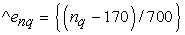(12)

Runner size adjustment to peak efficiency:(13)

Turbine peak efficiency:(14)

where:

Rm = turbine manufacture/design coefficient

for Kaplan turbines:

Peak efficiency flow:(15)

Efficiencies at flows above and below peak efficiency flow: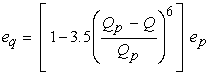(16)

for Propeller turbines:

Peak efficiency flow: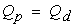(17)

Efficiencies at flows below peak efficiency flow: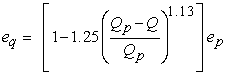(18)

for Pelton turbines:

Rotational speed: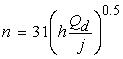(19)

i = number of jets (user – selected value from 1-6)

Outside diameter of runner: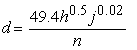(20)

Turbine peak efficiency:(21)

Peak efficiency flow:(22)

Efficiencies at flows above and below peak efficiency flow:(23)

for Turgo turbines:(24)

for Cross flow turbines:

Peak efficiency flow: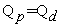Efficiency:(25)

All these formulae were written into codes and during runtime the values they generate are sent to the home form for them to be accessed by other forms, the generator efficiency is stored as well.

2.2.2. The Plant Capacity

The computed capacity of the plant is done using the following equations :(26)

where:The power duration values are computed using each available flow duration value in the equation as Q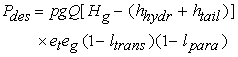(27)

where: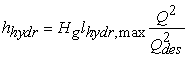(28)

lhydr,max = maximum hydraulic losses specified by the user(29)The renewable energy available is the area under the power duration curve and it is computed using the 21 values of power duration in the formula :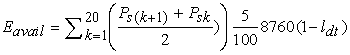(30)

where: ldt is the is annual downtme losses.

### 3. Results and Discussion

The program interface requires real data from a functioning hydropower plant to ascertain its correctness. At this stage of the development of this interface program, data from Small Hydropower Plants (SHPs) would have been appropriate, however data from SHPs in Nigeria are either difficult to come by or are not available at all. The data of Jebba hydropower plant, Nigeria were obtained and used for the testing . Although Jebba power plant is a large hydropower plant, using its data for testing is safe because the formulae for calculating the output of other sizes of hydropower plants are similar to that of small hydropower plant but additional losses need to be considered in the computation.

The input data used for the testing are:

i. The monthly flow data of the river Niger from 1984-2008

ii. Design flow of 380m3/s 

iv. Generator efficiency of 91%

v. Fixed blade (propeller) type turbine

vi. Latitude = 9.138, Longitude = 4.7883 .

The losses, residual flow and percentage specified for firm flow were however not available. Nonetheless the program interface was however tested without these values because although they are necessary for accuracy, estimation can be made without them, moreover subsequently assumptions of the unavailable values within acceptable range was used to show that if the values were known, the result would have been closer to the actual value.

With all the necessary input obtained, the program was run and tested. From the flow form shown in Figure 2 below, it can be seen that a flow of 3275 m3/s is obtainable from the site only at 1% of the time in a year; also a flow of 1880 m3/s is obtainable at 5% of the time and so on.

It can be seen from the Figure 3 that the flow values have been arranged in 5% increment of percentage equaled or exceeded, these are the values needed for further computation.

Because residual flow was not entered it was passed as 0 to the application and that was what was subtracted from the flow duration values, hence the same values for the flow duration are for the available flow duration as well.

3.1. Flow Duration Curve

The flow duration and available flow duration have the same line because they share the same value. The flow duration curve obtained is depicted in Figure 4 below.

The following data were assumed with restriction to their range.

Percentage specified = 50%

Residual flow = 200m3/s

Transformer losses = 0.0125

Parasitic electricity losses = 0.02021

Maximum hydraulic losses = 0.07

Maximum tail water effect = 1

Annual downtime losses = 0.04

3.2. The Power Duration Curve

The power values were equal except the last value because any value larger than the design flow will not be used, rather the design flow will be used in its stead, this is because the turbine cannot utilize more than it is designed to use. This application plotted the curve using the power duration values as shown in Figure 5, and the area under this curve is the renewable energy available.

As shown in Figure 6 below, a plant capacity of 96,400.77kW was obtained which is similar to the 96.4MW that a unit turbine generates in Jebba.

The performance of the program interface was satisfactory as it was able to compute the flow duration values and curve, available flow duration value and curve, plant capacity, power duration values and curve, renewable energy available etc. When compared with other commercially available computational interface for hydropower plants, such as the No Outage Com LLC interface output, it offers comparable details and it is user friendly like both RETScreen and No Outage Com LLC. However the program interface has some limitations which include its inability to be used to compute the energy demand for isolated grid.

### 4. Conclusion

Hydropower computation interface is essential for any hydropower installation and accurate estimation is needed for detailed information on the expected output of the plant. This program interface has the capability of computing the output of hydropower plants in a short time, develop a reliable expected flow and power characteristics of the site and generate a comprehensive report of the computation that is printable. The limitations of the program interface include its inability to compute the renewable energy delivered to an off grid load.

### References

  Castaldi, D.,Chastain, E., Windram, M., Ziatyk, L. ,(2003). A Study of Hydroelectric Power : From a Global Perspective to a Local Application .Accessed on 10/04/2012,available online at http://www.ems.psu.edu/vikingpaper pdf , pp 4-5.In article  Bennett, K., (1990), Clean Energy Project Analysis. Canada: Ret ScreenIn article  Wang Z., Hongliang H. (2012), Hydropower Computation Using Visual Basic for Application Programming. China: ICAPIE. Accessed on 12/04/2012, available online at http://www.sciencedirect.com.In article  Goran S. (2009), A Practical Guide to Assessment and Implementation of Small Hydropower.Australia: Tasmania. Accessed on 5/05/2012, available online at http://www.docstoc.com/docs/19906479/a-practical-guide-to-assesment-and-implementation-of-small , pp 1-5.In article  The Visual Studio Combined help collection, Microsoft Visual Studio 2008 Documentation, (2007), Microsoft CorporationIn article  A visual representation of how bubble sort works. Accessed on 20/12/2011, available online at http://en.m.wikipedia.org/wiki/bubble_sort.In article  Analysis Techniques: Flow Duration Analysis Tutorial (2002), Oregon State University. Accessed on 08/12/2011, available online at http://water.oregonstate.edu/streamflow/.In article  RETScreen® International Clean Energy Decision Support Centre (1997), RETScreen® Software Online User Manuals. Canada: Ret Screen.In article  Olukanni, D. O. & Salami, A. W. (2008). Fitting probability distribution functions to reservoir inflow at hydropower dams in Nigeria. Journal of Environmental Hydrology, USA, Vol. 16 Paper 35, pp. 1-7.In article  Jebba Hydro Power Plant Brief History. Accessed on 12/11/2011, available online at http://jebbahydroelectricplc.org.In article  Ajao K.R. & Sule B.F. (2011). Reduction of Carbon Dioxide (CO2) in the Atmosphere, Hydropower as a Viable Renewable Energy Resource. Journal of Basic and Applied Scientific Research, TextRoad Publication, pp. 2127-2128.In article  Jebba Hydroelectric power plant Nigeria-Geo. Accessed on 12/03/2012, available online at http://globalenergyobservatory.org/geoid/42544.In article

### APPENDIX I

Flow form codes

Imports System.IO

Imports System.Text

Public Class FrmFlow

Public i As Integer

Private Sub Button3_Click(ByVal sender As System.Object, ByVal e As System.EventArgs) Handles Button3.Click

Dim openDialog As New OpenFileDialog openDialog.ShowDialog()

Dim fileName As String

fileName = openDialog.FileName.ToString()

Debug.WriteLine(fileName.ToString())

Dim builder As New StringBuilder

Dim singleChar As String

Dim intSingleChar As Integer

Dim itemlist As New ArrayList

Try

outWrite = File.OpenText(fileName.ToString())

outWrite2 = File.OpenText(fileName.ToString())

Catch ex As Exception

Beep()

End Try

'counting the entries

Try

While outWrite.Peek <> -1

singleChar = Chr(intSingleChar)

'' Debug.WriteLine(singleChar)

If (singleChar.ToString() = "1") Then

ElseIf (singleChar.ToString() = "2") Then

ElseIf (singleChar.ToString() = "3") Then

ElseIf (singleChar.ToString() = "4") Then

ElseIf (singleChar.ToString() = "5") Then

ElseIf (singleChar.ToString() = "6") Then

ElseIf (singleChar.ToString() = "7") Then

ElseIf (singleChar.ToString() = "8") Then

ElseIf (singleChar.ToString() = "9") Then

ElseIf (singleChar.ToString() = "0") Then

ElseIf (singleChar.ToString() = vbNewLine Or singleChar.ToString() = vbCr) Then

Else

FrmHome.counter += 1

End If

End While

Catch ex As Exception

Debug.WriteLine(ex.ToString())

End Try

Dim counter2 As Integer

counter2 = 0

Try

While outWrite2.Peek <> -1

singleChar = Chr(intSingleChar)

'' Debug.WriteLine(singleChar)

If (singleChar.ToString() = "1") Then

builder.Append("1")

ElseIf (singleChar.ToString() = "2") Then

builder.Append("2")

ElseIf (singleChar.ToString() = "3") Then

builder.Append("3")

ElseIf (singleChar.ToString() = "4") Then

builder.Append("4")

ElseIf (singleChar.ToString() = "5") Then

builder.Append("5")

ElseIf (singleChar.ToString() = "6") Then

builder.Append("6")

ElseIf (singleChar.ToString() = "7") Then

builder.Append("7")

ElseIf (singleChar.ToString() = "8") Then

builder.Append("8")

ElseIf (singleChar.ToString() = "9") Then

builder.Append("9")

ElseIf (singleChar.ToString() = "0") Then

builder.Append("0")

ElseIf (singleChar.ToString().Equals(vbNewLine) Or singleChar.ToString().Equals(vbCr) Or singleChar.ToString().Equals(vbCrLf)) Then

builder.Append("")

Else

FrmHome.arrayflow(counter2) = Double.Parse(builder.ToString())

counter2 += 1

builder.Clear()

End If

End While

'bubble sort

Dim counter3 As Integer

counter3 = 1

Dim k, l As Integer

For k = 0 To FrmHome.arrayflow.Count - 2

For l = k + 1 To FrmHome.arrayflow.Count - 1

If FrmHome.arrayflow(k) < FrmHome.arrayflow(l) Then

swap(FrmHome.arrayflow(k), FrmHome.arrayflow(l))

End If

Next

Next

Dim n, m As Integer

FrmHome.rank1(n) = 1

For n = 0 To FrmHome.arrayflow.Count - 2

For m = n + 1 To FrmHome.arrayflow.Count - 1

If FrmHome.arrayflow(m) = FrmHome.arrayflow(n) Then

FrmHome.rank1(m) = FrmHome.rank1(n)

Else

FrmHome.rank1(m) = m + 1

End If

Next

Next

counter2 += 1

i = 0

DataGridView1.Rows.Clear()

Do While (i < FrmHome.counter)

FrmHome.calc = FrmHome.rank1(i) / (FrmHome.counter + 1)

FrmHome.calc = FrmHome.calc * 100

DataGridView1.Rows.Add(New String() {i + 1, FrmHome.arrayflow(i), FrmHome.rank1(i), FrmHome.calc.ToString("0.000")})

Debug.WriteLine(builder.ToString())

Debug.WriteLine(i)

i += 1

Loop

Button3.Enabled = False

FrmFlowDuration.flowduration()

Catch ex As Exception

MsgBox("No/Wrong file selected", MsgBoxStyle.Exclamation, "Selection not Identified")

End Try

End Sub

Private Sub swap(ByRef a As Integer, ByRef b As Integer)

Dim temp As Integer

temp = a

a = b

b = temp

End Sub

Private Sub Button1_Click(ByVal sender As System.Object, ByVal e As System.EventArgs) Handles Button1.Click

Me.Hide()

End Sub

Private Sub FrmFlow_Load(ByVal sender As System.Object, ByVal e As System.EventArgs) Handles MyBase.Load

End Sub

FrmResidualFLow.Show()

Me.Hide()

FrmResidualFLow.Visible = False

FrmResidualFLow.ShowDialog(FrmHome)

End Sub

Private Sub DataGridView1_CellContentClick(ByVal sender As System.Object, ByVal e As System.Windows.Forms.DataGridViewCellEventArgs) Handles DataGridView1.CellContentClick

End Sub

End Class

Flow duration codes

Public Class FrmFlowDuration

Public Sub availflow()

FrmAvailFlow.DataGridView1.Rows.Clear()

Dim i As Integer

i = 0

Dim val As Double

val = 0

Do While (i <= 20)

Try

FrmHome.arrayavailflow(i) = (FrmHome.resultarray.Item(i) - FrmHome.ResFlow)

Catch ex As Exception

MsgBox("please open the flow duration window first", , "Unable to load Data")

Exit Sub

End Try

FrmAvailFlow.DataGridView1.Rows.Add(New String() {(i + 1), FrmHome.arrayavailflow(i).ToString("0.00"), val.ToString("0.0")})

i += 1

val += 5

Loop

End Sub

Public Sub flowduration()

DataGridView1.Rows.Clear()

Dim val As Double

Dim val4, val5, val6, val7 As Double

Dim i As Integer

i = 1

val = 0

Do While (i <= 21)

If val = 0 Then

val4 = FrmHome.calcArray.Item(0)

val5 = FrmHome.calcArray.Item(1)

val6 = FrmHome.arrayflow(0)

val7 = FrmHome.arrayflow(1)

FrmHome.result = ((val - val4) * (val7 - val6)) / (val5 - val4)

FrmHome.result = FrmHome.result + val6

ElseIf val = 100 Then

val4 = FrmHome.calcArray.Item(FrmHome.calcArray.Count - 1)

val5 = FrmHome.calcArray.Item(FrmHome.calcArray.Count - 2)

val6 = FrmHome.arrayflow(FrmHome.calcArray.Count - 1)

val7 = FrmHome.arrayflow(FrmHome.calcArray.Count - 2)

FrmHome.result = ((val - val5) * (val6 - val7)) / (val4 - val5)

FrmHome.result = FrmHome.result + val7

Else

Dim val3 As Integer

val3 = FrmHome.calcArray.IndexOf(val)

If val3 < 0 Then

For Each calc1 As Double In FrmHome.calcArray

If val > calc1 Then

val4 = FrmHome.calcArray.Item(FrmHome.calcArray.LastIndexOf(calc1))

val5 = FrmHome.calcArray.Item(FrmHome.calcArray.LastIndexOf(calc1) + 1)

val6 = FrmHome.arrayflow(FrmHome.calcArray.LastIndexOf(calc1) + 1)

val7 = FrmHome.arrayflow(FrmHome.calcArray.LastIndexOf(calc1) + 2)

Else

Exit For

End If

Next

FrmHome.result = ((val - val4) * (val7 - val6)) / (val5 - val4)

FrmHome.result = FrmHome.result + val6

Else

Dim val13 As Double

val13 = FrmHome.arrayflow(val3 + 1)

FrmHome.result = val13

End If

End If

i = i + 1

val = val + 5

Loop

availflow()

End Sub

Private Sub DataGridView1_CellContentClick(ByVal sender As System.Object, ByVal e As System.Windows.Forms.DataGridViewCellEventArgs) Handles DataGridView1.CellContentClick

End Sub

Private Sub FrmFlowDuration_Load(ByVal sender As System.Object, ByVal e As System.EventArgs) Handles MyBase.Load

flowduration()

End Sub

Private Sub Button1_Click(ByVal sender As System.Object, ByVal e As System.EventArgs) Handles Button1.Click

Me.Hide()

End Sub

End Class

Plant capacity codes

Public Class FrmPlantCapacity

Private Sub FrmPlantCapacity_Load(ByVal sender As System.Object, ByVal e As System.EventArgs) Handles MyBase.Load

Dim hhydr As Single

hhydr = FrmHome.grosshead * FrmHome.Lhydrmax * (FrmHome.desflow ^ 2 / FrmHome.desflow ^ 2)

'for francis turbine

Dim df As Single

Dim nqf As Single

Dim enqf As Single

Dim edf As Single

Dim epf As Single

Dim Qpf As Single

Dim depf As Single

Dim erf As Single

df = ((0.46) * ((FrmHome.desflow) ^ (0.473)))

nqf = 600 * ((FrmHome.grosshead - FrmHome.Lhydrmax) ^ (-0.5))

enqf = ((nqf - 56) / 256) ^ 2

edf = ((0.081 + enqf) * (1 - ((0.789) * (df ^ (-0.2)))))

epf = (0.919 - enqf + edf) - 0.0305 + (0.05 * 4.5)

Qpf = 0.65 * FrmHome.desflow * (nqf ^ (0.05))

depf = 0.007 * (nqf ^ (0.4))

erf = (1 - depf) * epf

'for kaplan turbine

Dim dk As Single

Dim nqk As Single

Dim enqk As Single

Dim edk As Single

Dim epk As Single

Dim Qpk As Single

dk = ((0.46) * ((FrmHome.desflow) ^ (0.473)))

nqk = 800 * ((FrmHome.grosshead - FrmHome.Lhydrmax) ^ (-0.5))

enqk = ((nqk - 170) / 700) ^ 2

edk = ((0.095 + enqk) * (1 - ((0.789) * (dk ^ (-0.2)))))

epk = (0.905 - enqk + edk) - 0.0305 + (0.05 * 4.5)

Qpk = 0.75 * FrmHome.desflow

'propellor turbine

Dim dp As Single

Dim nqp As Single

Dim enqp As Single

Dim edp As Single

Dim epp As Single

Dim Qpp As Single

dp = ((0.46) * ((FrmHome.desflow) ^ (0.473)))

nqp = 800 * ((FrmHome.grosshead - FrmHome.Lhydrmax) ^ (-0.5))

enqp = ((nqk - 170) / 700) ^ 2

edp = ((0.095 + enqp) * (1 - ((0.789) * (dp ^ (-0.2)))))

epp = (0.905 - enqp + edp) - 0.0305 + (0.05 * 4.5)

Qpp = FrmHome.desflow

'for pelton turbine

Dim npel As Single

Dim dpel As Single

Dim eppel As Single

Dim Qppel As Single

npel = 31 * (((FrmHome.grosshead - FrmHome.Lhydrmax) * (FrmHome.desflow / FrmHome.j)) ^ (0.5))

dpel = (49.4 * ((FrmHome.grosshead - FrmHome.Lhydrmax) ^ (0.5)) * ((FrmHome.j) ^ (0.02))) / npel

eppel = 0.864 * (dpel ^ (0.04))

Qppel = (0.662 + (0.001 * FrmHome.j)) * FrmHome.desflow

'For turgo

Dim ept As Single

ept = eppel - 0.03

'For crossflow

Dim Qpc As Single

Qpc = FrmHome.desflow

'manual plant capacity

FrmHome.Pdes = (1000 * 9.81 * FrmHome.desflow) * (FrmHome.grosshead) * (1 - FrmHome.Lhydrmax) * (FrmHome.eff) * FrmHome.Eg * (1 - FrmHome.Ltrans) * (1 - FrmHome.Lpara)

End If

'francis plant capacity

Dim eff As Single

If FrmHome.desflow = Qpf Then

eff = epf

ElseIf FrmHome.desflow < Qpf Then

eff = (1 - (1.25 * (((Qpf - FrmHome.desflow) / Qpf) ^ (3.94 - 0.0195 * nqf)))) * epf

ElseIf FrmHome.desflow > Qpf Then

eff = epf - ((((FrmHome.desflow - Qpf) / (FrmHome.desflow - Qpf)) ^ 2) * (epf - erf))

End If

FrmHome.Pdes = (1000 * 9.81 * FrmHome.desflow) * (FrmHome.grosshead) * (1 - FrmHome.Lhydrmax) * (eff) * FrmHome.Eg * (1 - FrmHome.Ltrans) * (1 - FrmHome.Lpara)

End If

'kaplan plant capacity

Dim eff As Single

If FrmHome.desflow = Qpk Then

eff = epk

Else

eff = (1 - 3.5 * (((Qpk - FrmHome.desflow) / Qpk) ^ 6)) * epk

End If

FrmHome.Pdes = (1000 * 9.81 * FrmHome.desflow) * (FrmHome.grosshead) * (1 - FrmHome.Lhydrmax) * (eff) * FrmHome.Eg * (1 - FrmHome.Ltrans) * (1 - FrmHome.Lpara)

End If

'propellor plant capacity

Dim eff As Single

eff = epp

FrmHome.Pdes = (1000 * 9.81 * FrmHome.desflow) * (FrmHome.grosshead) * (1 - FrmHome.Lhydrmax) * (eff) * FrmHome.Eg * (1 - FrmHome.Ltrans) * (1 - FrmHome.Lpara)

End If

Dim eff As Single

If FrmHome.desflow = Qppel Then

eff = eppel

Else

eff = (1 - ((1.31 + (0.025 * FrmHome.j)) * (Math.Abs((Qppel - FrmHome.desflow) / Qppel)) ^ (5.6 + (0.4 * FrmHome.j)))) * eppel

End If

FrmHome.Pdes = (1000 * 9.81 * FrmHome.desflow) * (FrmHome.grosshead) * (1 - FrmHome.Lhydrmax) * (eff) * FrmHome.Eg * (1 - FrmHome.Ltrans) * (1 - FrmHome.Lpara)

End If

Dim eff As Single

If FrmHome.desflow = Qppel Then

eff = ept

Else

eff = ((1 - ((1.31 + (0.025 * FrmHome.j)) * (Math.Abs((Qppel - FrmHome.desflow) / Qppel)) ^ (5.6 + (0.4 * FrmHome.j)))) * eppel) - 0.03

End If

FrmHome.Pdes = (1000 * 9.81 * FrmHome.desflow) * (FrmHome.grosshead) * (1 - FrmHome.Lhydrmax) * (eff) * FrmHome.Eg * (1 - FrmHome.Ltrans) * (1 - FrmHome.Lpara)

End If

Dim eff As Single

eff = 0.79 - 0.15 * ((FrmHome.desflow - FrmHome.desflow) / Qpc) - 1.37 * (((FrmHome.desflow - FrmHome.desflow) / Qpc) ^ 14)

FrmHome.Pdes = (1000 * 9.81 * FrmHome.desflow) * (FrmHome.grosshead) * (1 - FrmHome.Lhydrmax) * (eff) * FrmHome.Eg * (1 - FrmHome.Ltrans) * (1 - FrmHome.Lpara)

End If

If FrmHome.Pdes = 0 Then

Label1.Text = "One or some of the parameters needed for this computation has not been entered, go to help for assistance"

Else

FrmHome.Pdes = FrmHome.Pdes / 1000

Label1.Text = "The Plant has the capacity of " & FrmHome.Pdes.ToString("#,###.##") & " " & "kW"

Frmpowerduration.powerduration()

End If

End Sub

Private Sub Button1_Click(ByVal sender As Object, ByVal e As System.EventArgs) Handles Button1.Click

Me.Hide()

End Sub

End Class

Power duration codes

Public Class Frmpowerduration

Public Sub powerduration()

Dim hhydr, htail As Single

DataGridView1.Rows.Clear()

Dim c As Integer

'for francis turbine

Dim df As Single

Dim nqf As Single

Dim enqf As Single

Dim edf As Single

Dim epf As Single

Dim Qpf As Single

Dim depf As Single

Dim erf As Single

df = ((0.46) * ((FrmHome.desflow) ^ (0.473)))

nqf = 600 * ((FrmHome.grosshead - FrmHome.Lhydrmax) ^ (-0.5))

enqf = ((nqf - 56) / 256) ^ 2

edf = ((0.081 + enqf) * (1 - ((0.789) * (df ^ (-0.2)))))

epf = (0.919 - enqf + edf) - 0.0305 + (0.05 * 4.5)

Qpf = 0.65 * FrmHome.desflow * (nqf ^ (0.05))

depf = 0.007 * (nqf ^ (0.4))

erf = (1 - depf) * epf

'for kaplan turbine

Dim dk As Single

Dim nqk As Single

Dim enqk As Single

Dim edk As Single

Dim epk As Single

Dim Qpk As Single

dk = ((0.46) * ((FrmHome.desflow) ^ (0.473)))

nqk = 800 * ((FrmHome.grosshead - FrmHome.Lhydrmax) ^ (-0.5))

enqk = ((nqk - 170) / 700) ^ 2

edk = ((0.095 + enqk) * (1 - ((0.789) * (dk ^ (-0.2)))))

epk = (0.905 - enqk + edk) - 0.0305 + (0.05 * 4.5)

Qpk = 0.75 * FrmHome.desflow

'propellor turbine

Dim dp As Single

Dim nqp As Single

Dim enqp As Single

Dim edp As Single

Dim epp As Single

Dim Qpp As Single

dp = ((0.46) * ((FrmHome.desflow) ^ (0.473)))

nqp = 800 * ((FrmHome.grosshead - FrmHome.Lhydrmax) ^ (-0.5))

enqp = ((nqk - 170) / 700) ^ 2

edp = ((0.095 + enqp) * (1 - ((0.789) * (dp ^ (-0.2)))))

epp = (0.905 - enqp + edp) - 0.0305 + (0.005 * 4.5)

Qpp = FrmHome.desflow

'for pelton turbine

Dim npel As Single

Dim dpel As Single

Dim eppel As Single

Dim Qppel As Single

npel = 31 * (((FrmHome.grosshead - FrmHome.Lhydrmax) * (FrmHome.desflow / FrmHome.j)) ^ (0.5))

dpel = (49.4 * ((FrmHome.grosshead - FrmHome.Lhydrmax) ^ (0.5)) * ((FrmHome.j) ^ (0.02))) / npel

eppel = 0.864 * (dpel ^ (0.04))

Qppel = (0.662 + (0.001 * FrmHome.j)) * FrmHome.desflow

'For turgo

Dim ept As Single

ept = eppel - 0.03

'For crossflow

Dim Qpc As Single

Qpc = FrmHome.desflow

'manual power duration

For c = 0 To 20

If FrmHome.arrayavailflow(c) > FrmHome.desflow Then

htail = FrmHome.Htailmax * (((FrmHome.resultarray.Item(c) - FrmHome.desflow) ^ 2) / ((FrmHome.arrayflow(0) - FrmHome.desflow) ^ 2))

FrmHome.arrayavailflow(c) = FrmHome.desflow

hhydr = FrmHome.grosshead * FrmHome.Lhydrmax * (FrmHome.desflow ^ 2 / FrmHome.desflow ^ 2)

ElseIf FrmHome.arrayavailflow(c) < FrmHome.desflow Then

FrmHome.arrayavailflow(c) = FrmHome.arrayavailflow(c)

hhydr = FrmHome.grosshead * FrmHome.Lhydrmax * ((FrmHome.arrayavailflow(c)) ^ 2 / FrmHome.desflow ^ 2)

htail = 0

End If

FrmHome.pduration(c) = (1000 * 9.81 * FrmHome.arrayavailflow(c)) * ((FrmHome.grosshead) - (hhydr + htail)) * (FrmHome.eff) * FrmHome.Eg * (1 - FrmHome.Ltrans) * (1 - FrmHome.Lpara)

Next

End If

'francis plant capacity

Dim eff As Single

For c = 0 To 20

If FrmHome.arrayavailflow(c) > FrmHome.desflow Then

htail = FrmHome.Htailmax * (((FrmHome.resultarray.Item(c) - FrmHome.desflow) ^ 2) / ((FrmHome.arrayflow(0) - FrmHome.desflow) ^ 2))

FrmHome.arrayavailflow(c) = FrmHome.desflow

hhydr = FrmHome.grosshead * FrmHome.Lhydrmax * (FrmHome.desflow ^ 2 / FrmHome.desflow ^ 2)

ElseIf FrmHome.arrayavailflow(c) < FrmHome.desflow Then

FrmHome.arrayavailflow(c) = FrmHome.arrayavailflow(c)

hhydr = FrmHome.grosshead * FrmHome.Lhydrmax * ((FrmHome.arrayavailflow(c)) ^ 2 / FrmHome.desflow ^ 2)

htail = 0

End If

If FrmHome.arrayavailflow(c) = Qpf Then

eff = epf

ElseIf FrmHome.arrayavailflow(c) < Qpf Then

eff = (1 - (1.25 * (((Qpf - FrmHome.arrayavailflow(c)) / Qpf) ^ (3.94 - 0.0195 * nqf)))) * epf

ElseIf FrmHome.arrayavailflow(c) > Qpf Then

eff = epf - ((((FrmHome.arrayavailflow(c) - Qpf) / (FrmHome.desflow - Qpf)) ^ 2) * (epf - erf))

End If

FrmHome.pduration(c) = (1000 * 9.81 * FrmHome.arrayavailflow(c)) * ((FrmHome.grosshead) - (hhydr + htail)) * (eff) * FrmHome.Eg * (1 - FrmHome.Ltrans) * (1 - FrmHome.Lpara)

Next

End If

'kaplan plant capacity

Dim eff As Single

For c = 0 To 20

If FrmHome.arrayavailflow(c) > FrmHome.desflow Then

htail = FrmHome.Htailmax * (((FrmHome.resultarray.Item(c) - FrmHome.desflow) ^ 2) / ((FrmHome.arrayflow(0) - FrmHome.desflow) ^ 2))

FrmHome.arrayavailflow(c) = FrmHome.desflow

hhydr = FrmHome.grosshead * FrmHome.Lhydrmax * (FrmHome.desflow ^ 2 / FrmHome.desflow ^ 2)

ElseIf FrmHome.arrayavailflow(c) < FrmHome.desflow Then

FrmHome.arrayavailflow(c) = FrmHome.arrayavailflow(c)

hhydr = FrmHome.grosshead * FrmHome.Lhydrmax * ((FrmHome.arrayavailflow(c)) ^ 2 / FrmHome.desflow ^ 2)

htail = 0

End If

If FrmHome.arrayavailflow(c) = Qpk Then

eff = epk

Else

eff = (1 - 3.5 * (((Qpk - FrmHome.desflow) / Qpk) ^ 6)) * epk

End If

FrmHome.pduration(c) = (1000 * 9.81 * FrmHome.arrayavailflow(c)) * ((FrmHome.grosshead) - (hhydr + htail)) * (eff) * FrmHome.Eg * (1 - FrmHome.Ltrans) * (1 - FrmHome.Lpara)

Next

End If

'propellor plant capacity

Dim eff As Single

For c = 0 To 20

If FrmHome.arrayavailflow(c) > FrmHome.desflow Then

htail = FrmHome.Htailmax * (((FrmHome.resultarray.Item(c) - FrmHome.desflow) ^ 2) / ((FrmHome.arrayflow(0) - FrmHome.desflow) ^ 2))

FrmHome.arrayavailflow(c) = FrmHome.desflow

hhydr = FrmHome.grosshead * FrmHome.Lhydrmax * (FrmHome.desflow ^ 2 / FrmHome.desflow ^ 2)

ElseIf FrmHome.arrayavailflow(c) < FrmHome.desflow Then

FrmHome.arrayavailflow(c) = FrmHome.arrayavailflow(c)

hhydr = FrmHome.grosshead * FrmHome.Lhydrmax * ((FrmHome.arrayavailflow(c)) ^ 2 / FrmHome.desflow ^ 2)

htail = 0

End If

If FrmHome.arrayavailflow(c) = Qpp Then

eff = epp

Else

eff = (1 - 1.25 * ((Qpp - FrmHome.arrayavailflow(c)) / Qpp) ^ 1.13) * epp

End If

FrmHome.pduration(c) = (1000 * 9.81 * FrmHome.arrayavailflow(c)) * ((FrmHome.grosshead) - (hhydr + htail)) * (eff) * FrmHome.Eg * (1 - FrmHome.Ltrans) * (1 - FrmHome.Lpara)

Next

End If

Dim eff As Single

For c = 0 To 20

If FrmHome.arrayavailflow(c) > FrmHome.desflow Then

htail = FrmHome.Htailmax * (((FrmHome.resultarray.Item(c) - FrmHome.desflow) ^ 2) / ((FrmHome.arrayflow(0) - FrmHome.desflow) ^ 2))

FrmHome.arrayavailflow(c) = FrmHome.desflow

hhydr = FrmHome.grosshead * FrmHome.Lhydrmax * (FrmHome.desflow ^ 2 / FrmHome.desflow ^ 2)

ElseIf FrmHome.arrayavailflow(c) < FrmHome.desflow Then

FrmHome.arrayavailflow(c) = FrmHome.arrayavailflow(c)

hhydr = FrmHome.grosshead * FrmHome.Lhydrmax * ((FrmHome.arrayavailflow(c)) ^ 2 / FrmHome.desflow ^ 2)

htail = 0

End If

If FrmHome.desflow = Qppel Then

eff = eppel

Else

eff = (1 - ((1.31 + (0.025 * FrmHome.j)) * (Math.Abs((Qppel - FrmHome.desflow) / Qppel)) ^ (5.6 + (0.4 * FrmHome.j)))) * eppel

End If

FrmHome.pduration(c) = (1000 * 9.81 * FrmHome.arrayavailflow(c)) * ((FrmHome.grosshead) - (hhydr + htail)) * (eff) * FrmHome.Eg * (1 - FrmHome.Ltrans) * (1 - FrmHome.Lpara)

Next

End If

Dim eff As Single

For c = 0 To 20

If FrmHome.arrayavailflow(c) > FrmHome.desflow Then

htail = FrmHome.Htailmax * (((FrmHome.resultarray.Item(c) - FrmHome.desflow) ^ 2) / ((FrmHome.arrayflow(0) - FrmHome.desflow) ^ 2))

FrmHome.arrayavailflow(c) = FrmHome.desflow

hhydr = FrmHome.grosshead * FrmHome.Lhydrmax * (FrmHome.desflow ^ 2 / FrmHome.desflow ^ 2)

ElseIf FrmHome.arrayavailflow(c) < FrmHome.desflow Then

FrmHome.arrayavailflow(c) = FrmHome.arrayavailflow(c)

hhydr = FrmHome.grosshead * FrmHome.Lhydrmax * ((FrmHome.arrayavailflow(c)) ^ 2 / FrmHome.desflow ^ 2)

htail = 0

End If

If FrmHome.desflow = Qppel Then

eff = ept

Else

eff = ((1 - ((1.31 + (0.025 * FrmHome.j)) * (Math.Abs((Qppel - FrmHome.desflow) / Qppel)) ^ (5.6 + (0.4 * FrmHome.j)))) * eppel) - 0.03

End If

FrmHome.pduration(c) = (1000 * 9.81 * FrmHome.arrayavailflow(c)) * ((FrmHome.grosshead) - (hhydr + htail)) * (eff) * FrmHome.Eg * (1 - FrmHome.Ltrans) * (1 - FrmHome.Lpara)

Next

End If

Dim eff As Single

For c = 0 To 20

If FrmHome.arrayavailflow(c) > FrmHome.desflow Then

htail = FrmHome.Htailmax * (((FrmHome.resultarray.Item(c) - FrmHome.desflow) ^ 2) / ((FrmHome.arrayflow(0) - FrmHome.desflow) ^ 2))

FrmHome.arrayavailflow(c) = FrmHome.desflow

hhydr = FrmHome.grosshead * FrmHome.Lhydrmax * (FrmHome.desflow ^ 2 / FrmHome.desflow ^ 2)

ElseIf FrmHome.arrayavailflow(c) < FrmHome.desflow Then

FrmHome.arrayavailflow(c) = FrmHome.arrayavailflow(c)

hhydr = FrmHome.grosshead * FrmHome.Lhydrmax * ((FrmHome.arrayavailflow(c)) ^ 2 / FrmHome.desflow ^ 2)

htail = 0

End If

eff = 0.79 - 0.15 * ((FrmHome.desflow - FrmHome.desflow) / Qpc) - 1.37 * (((FrmHome.desflow - FrmHome.desflow) / Qpc) ^ 14)

FrmHome.pduration(c) = (1000 * 9.81 * FrmHome.arrayavailflow(c)) * ((FrmHome.grosshead) - (hhydr + htail)) * (eff) * FrmHome.Eg * (1 - FrmHome.Ltrans) * (1 - FrmHome.Lpara)

Next

End If

'populating the datagrid

Dim d, value As Integer

d = 0

value = 0

Do While (d <= 20)

FrmHome.pduration(d) = FrmHome.pduration(d) / 1000

DataGridView1.Rows.Add(New String() {(d + 1), FrmHome.pduration(d).ToString("#,###.##"), value})

d += 1

value += 5

Loop

End Sub

Private Sub Frmpowerduration_Load(ByVal sender As System.Object, ByVal e As System.EventArgs) Handles MyBase.Load

If FrmHome.arrayavailflow(0) = 0 Then

Dim result As DialogResult = MessageBox.Show("Power duration can not be computed because flow values have not been loaded. Do you want to load flow data?", _

"Unable to compute power duration", MessageBoxButtons.YesNo, MessageBoxIcon.Question, MessageBoxDefaultButton.Button1)

If result = DialogResult.Yes Then

Me.Close()

FrmFlow.ShowDialog()

ElseIf result = DialogResult.No Then

Me.Close()

End If

Else

powerduration()

End If

End Sub

Private Sub Button1_Click(ByVal sender As System.Object, ByVal e As System.EventArgs) Handles Button1.Click

Me.Hide()

End Sub

End Class

Renewable energy available codes

Public Class FrmRenEnergy

Private Sub RenEnergy_Load(ByVal sender As System.Object, ByVal e As System.EventArgs) Handles MyBase.Load

If FrmHome.arrayavailflow(0) = 0 Then

Dim result As DialogResult = MessageBox.Show("Renewable energy available can not be computed because flow values have not been loaded. Do you want to load flow data?", _

"Unable to compute Renewable energy available", MessageBoxButtons.YesNo, MessageBoxIcon.Question, MessageBoxDefaultButton.Button1)

If result = DialogResult.Yes Then

Me.Close()

FrmFlow.ShowDialog()

ElseIf result = DialogResult.No Then

Me.Close()

End If

Else

Dim k As Integer

Dim Db, Dd, Dc As Double

k = 0

Db = 0

Do While (k <= 19)

Dd = ((FrmHome.pduration(k) + (FrmHome.pduration(k + 1))) / 2) * (5 / 100) * 8760 * (1 - FrmHome.Ldt)

Dc = Dd + Db

Db = Dc

k += 1

Loop

FrmHome.RenEnergy = Db

Db = Db / 1000

Label1.Text = ("The Renewable Energy Available is " & Db.ToString("#,###.##") & " kWh/yr")

End If

End Sub

Private Sub Button1_Click(ByVal sender As System.Object, ByVal e As System.EventArgs) Handles Button1.Click

Me.Hide()

End Sub

End Class